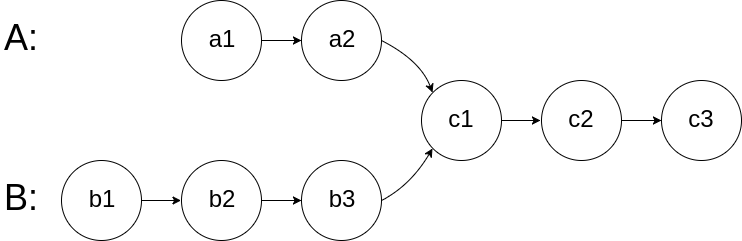New update is available. Click here to update.

# Intersection of Two Linked Lists

Last Updated: 22 Jul, 2020
Difficulty: Easy

## PROBLEM STATEMENT

#### For example:-

``````The given Linked Lists, where a1, a2, c1 is the first linked list, b1, b2, b3, c1 is the second linked list, and c1, c2, c3 is the third linked list which are merging at node c1.
``````##### Input Format:
``````The input format contains three lines consisting of three singly-linked lists.

All three lines contain the elements of the singly linked list separated by a single space and terminated by -1.

So first linked list would contain
a1, a2, ...an, c1, -1.

Similarly, the second line would contain
b1,b2, ...bm, c1, -1.

The third line would contain
c2, c3, ....ck, -1.
``````
##### Output format :
``````The only line of output contains data of the first merged node. If there is no merging output should contain -1.

You don't have to explicitly print by yourself. It has been taken care of.
``````
##### Constraints :
``````0 <= N <= 10^5
0 <= M <= 10^5
0 <= K <= 10^5.
-10^9 <= data <= 10^9 and data != -1

Time Limit: 1 sec
``````